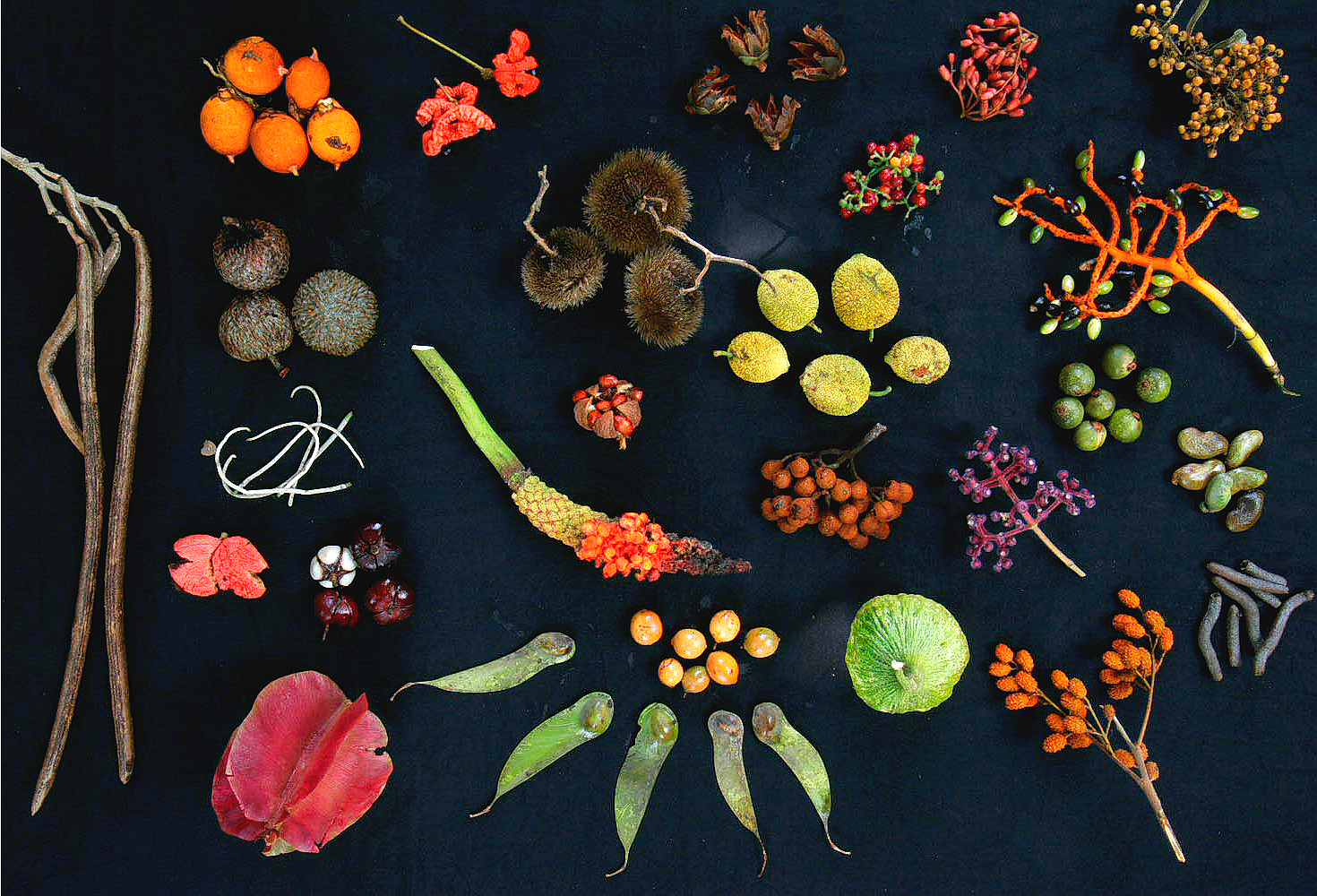## Biology as Information DynamicsIf biology is the study of self-replicating entities, and we want to understand the role of information, it makes sense to see how information theory is connected to the 'replicator equation' — a simple model of population dynamics for self-replicating entities. The relevant concept of information turns out to be the information of one probability distribution relative to another, also known as the Kullback–Leibler divergence. Using this we can get a new outlook on free energy, see evolution as a learning process, and give a clearer, more general formulation of Fisher's fundamental theorem of natural selection.

You can see the the slides for this talk, and also this video:

In the video there's a typo which I fixed later in the slides: $$\exp(-kE_i/T)$$ should be $$\exp(-E_i/kT)$$. For more, read:

The last reference contains proofs of the equations shown in red in my slides. In particular, Part 16 contains a proof of my updated version of Fisher's fundamental theorem.
© 2017 John Baez except the photo is by Christian Ziegler.
baez@math.removethis.ucr.andthis.edu